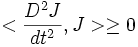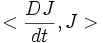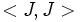# Nonpositively curved implies conjugate-free

## Statement

### Verbal statement

If the sectional curvature of a Riemannian manifold is everywhere nonpositive, then the Riemannian manifold is conjugate-free, viz it does not contain a pair of conjugate points.

## Proof

Let$M$ be the Riemannian manifold,$\gamma$ a geodesic in$M$ and$J$ a Jacobi field about$\gamma$ that vanishes at the endpoints. We must show that$J$ is everywhere zero.

Consider the Jacobi equation:$\frac{D^2J}{dt^2} + R(J,V)V = 0$

Taking the inner product of both these with$J$, the second term becomes the sectional curvature of the tangent plane spanned by$J$ and$V$, which is negative. Hence we conclude that:$<\frac{D^2J}{dt^2},J> \ge 0$

This tells us that$<\frac{DJ}{dt},J>$ is nondecreasing, and hence, that$$ is nondecreasing. Thus$J$ cannot be zero at both endpoints unless it is identically zero.

## Application

This observation is crucial to the proof of the Cartan-Hadamard theorem.